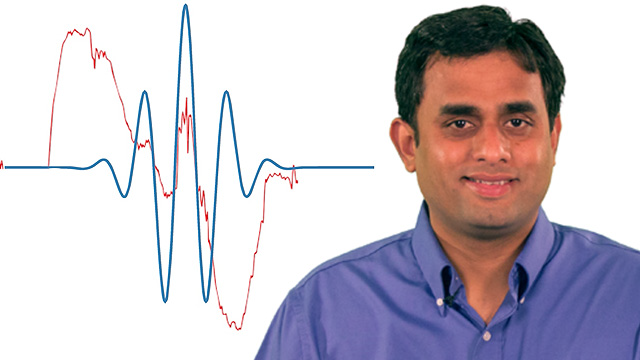# Get Started with Wavelet Toolbox

Analyze and synthesize signals and images using wavelets

Wavelet Toolbox™ provides functions and apps for analyzing and synthesizing signals and images. The toolbox includes algorithms for continuous wavelet analysis, wavelet coherence, synchrosqueezing, and data-adaptive time-frequency analysis. The toolbox also includes apps and functions for decimated and nondecimated discrete wavelet analysis of signals and images, including wavelet packets and dual-tree transforms.

Using continuous wavelet analysis, you can study the way spectral features evolve over time, identify common time-varying patterns in two signals, and perform time-localized filtering. Using discrete wavelet analysis, you can analyze signals and images at different resolutions to detect changepoints, discontinuities, and other events not readily visible in raw data. You can compare signal statistics on multiple scales, and perform fractal analysis of data to reveal hidden patterns.

With Wavelet Toolbox you can obtain a sparse representation of data, useful for denoising or compressing the data while preserving important features. Many toolbox functions support C/C++ code generation for desktop prototyping and embedded system deployment.

## VideosUnderstanding Wavelets, Part 1: What Are Wavelets
Explore the fundamental concepts of wavelet transforms in this introductory MATLAB Tech Talk. This video covers what wavelets are and how you can use them to explore your data in MATLAB. The video focuses on two important wavelet transform concepts: scaling and shifting. The concepts can be applied to 2-D data such as images.Understanding Wavelets, Part 2: Types of Wavelet Transforms
Explore the workings of wavelet transforms in detail. You will learn more about the continuous wavelet transforms and the discrete wavelet transform. You will also learn important applications of using wavelet transforms with MATLAB.Understand Wavelets, Part 3: An Example Application of the Discrete Wavelet Transform
Learn how to use to wavelets to denoise a signal while preserving its sharp features in this MATLAB Tech Talk. This video outlines the steps involved in denoising a signal with the discrete wavelet transform using MATLAB. Learn how this denoising technique compares with other denoising techniques.Understanding Wavelets, Part 4: An Example Application of the Continuous Wavelet Transform
Explore a practical application of using continuous wavelet transforms in this MATLAB Tech Talk. Get an overview of how to use MATLAB to obtain a sharper time-frequency analysis of a signal with the continuous wavelet transform. This video uses an example seismic signal to highlight the frequency localization capabilities of the continuous wavelet transform.

## SupportGet trial now